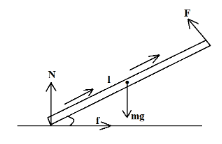QuestionAnswers

# The minimum coefficient of friction ${{\mu }_{\min }}$ between a thin homogeneous rod and a floor at which a person can slowly lift the rod from the floor without slippage to the vertical position, applying to it a force perpendicular to it is $\dfrac{1}{x\sqrt{x}}$. Find the value of $x$.Hint: We can find the value of coefficient of friction by finding the ratio of the resistive force and the value of normal or perpendicular force. The force of friction always acts in the direction opposite to the direction of intended or actual motion.

Formula used:
$T=Fr\sin \theta$
Where $T$is the torque, $F$ is the force, $r$ is the radius and $\theta$ is the angle between $F$ and lever arm

Coefficient of friction is described as the ratio of the force of friction between two bodies and the force pressing them together. It is a value that represents the relationship between two objects and the normal force or reaction between the objects. Coefficient of friction is of two types, Static and Kinetic coefficient of friction. Static coefficient of friction is applied to the stationary bodies while Kinetic coefficient is applied to the bodies in motion. Stationary or motionless objects experience more friction than those which are already in motion. We can say that it requires more force to put an object in motion than to sustain them in motion.
In the above question we are given that the rod does not slip on the floor, meaning we can apply the equations to equate all the forces as the rod is in equilibrium.
The forces acting on the rod are – gravitational force, applied force and the torque about the point of intersection of gravitational and applied force.\begin{align} & \text{Gravitational force = }mg \\ & \text{Applied force = }F \\ & \text{Torque = }T \\ & \text{Angle of torque = }\alpha \\ \end{align}

Balancing all the forces and torque acting on the rod,

$\sum\limits_{{}}^{{}}{T=Nl\cos \alpha -fl\left( \dfrac{1}{\sin \alpha }+\sin \alpha \right)}=0$
Where $N$i s the normal force and $f$ is the force of friction

The moment arm for normal force $N$ is $l\cos \alpha$ while for friction it is $l\left( \dfrac{1}{\sin \alpha }+\sin \alpha \right)$
From above equation we get,

\begin{align} & f=N\left( \dfrac{\cos \alpha \times \sin \alpha }{1+{{\sin }^{2}}\alpha } \right)=N\left( \dfrac{\cos \alpha \times \sin \alpha }{2{{\sin }^{2}}\alpha +{{\cos }^{2}}\alpha } \right) \\ & f=N\left( \dfrac{\cos \alpha \times \sin \alpha }{2\tan \alpha +\cot \alpha } \right) \\ \end{align}

Also, the force of friction cannot exceed $\mu N$, we have

$\mu \ge \dfrac{1}{2\tan \alpha +\cot \alpha }$

For minimum value of$\mu$, $2\tan \alpha +\cot \alpha$should be maximum

\begin{align} & \dfrac{d(2\tan \alpha +\cot \alpha )}{dx}=0 \\ & 2{{\sec }^{2}}\alpha -\cos e{{c}^{2}}\alpha =0 \\ & \tan \alpha =\dfrac{1}{\sqrt{2}} \\ \end{align}
We get$\mu =\dfrac{1}{2\sqrt{2}}$
Comparing with$\dfrac{1}{x\sqrt{x}}$,
Value of $x=2$

Note:
While calculating the torque, always choose a point where maximum lines of forces are passing so that the number of resultant torques will be minimum. For no confusion, choose clockwise direction as positive and anti-clockwise as negative.

View Notes
Relationship Between Force of Limiting Friction and Normal ReactionStatic FrictionRolling Friction and Rolling ResistanceCBSE Class 11 Physics Law of Motion FormulasCBSE Class 11 Physics Thermal Properties of Matter FormulasCBSE Class 11 Physics Mechanical Properties of Solids FormulasCBSE Class 11 Physics Mechanical Properties of Fluids FormulasCoefficient of DeterminationCBSE Class 11 Physics Systems of Particles and Rotational Motion FormulasKarl Pearson’s Coefficient of CorrelationImportant Questions for CBSE Class 11 Physics Chapter 11 - Thermal Properties of MatterImportant Questions for CBSE Class 11 Physics Chapter 5 - Law of MotionImportant Questions for CBSE Class 11 Physics Chapter 9 - Mechanical Properties of SolidsImportant Questions for CBSE Class 11 Physics Chapter 10 - Mechanical Properties of FluidsCBSE Class 8 Science Friction WorksheetsImportant Questions for CBSE Class 11 PhysicsImportant Questions for CBSE Class 11 Physics Chapter 7 - Systems of Particles and Rotational MotionImportant Questions for CBSE Class 12 Physics Chapter 11 - Dual Nature of Radiation and MatterImportant Questions for CBSE Class 11 English Snapshots Chapter 1 - The Summer of the Beautiful White HorseImportant Questions for CBSE Class 11 Biology Chapter 8 - Cell The Unit of LifeCBSE Class 12 Physics Question Paper 2020Previous Year Question Paper for CBSE Class 12 PhysicsPrevious Year Question Paper for CBSE Class 12 Physics - 2015Previous Year Question Paper for CBSE Class 12 Physics - 2018Previous Year Question Paper for CBSE Class 12 Physics - 2014Previous Year Question Paper for CBSE Class 12 Physics - 2013Previous Year Question Paper for CBSE Class 12 Physics - 2019Previous Year Physics Question Paper for CBSE Class 12 - 2017Physics Question Paper for CBSE Class 12 - 2016 Set 1 CPrevious Year Question Paper of CBSE Class 10 EnglishHC Verma Class 11 Physics Part-1 Solutions for Chapter 6 - FrictionNCERT Exemplar for Class 11 Physics Chapter 11 - Thermal Properties of Matter (Book Solutions)NCERT Solutions for Class 11 Physics Chapter 11 Thermal Properties of Matter in HindiFriction NCERT Solutions - Class 8 ScienceClass 11 Physics NCERT solutionsNCERT Solutions for Class 11 Physics Chapter 5 Law of Motion in HindiNCERT Exemplar for Class 11 Physics Chapter 10 - Mechanical Properties of Fluids (Book Solutions)NCERT Exemplar for Class 11 Physics Chapter 5 - Law of Motion (Book Solutions)NCERT Solutions for Class 11 Physics Chapter 10 Mechanical Properties of Fluids in HindiNCERT Solutions Class 11 English Woven Words Prose Chapter 4 The Adventure of the Three Garridebs